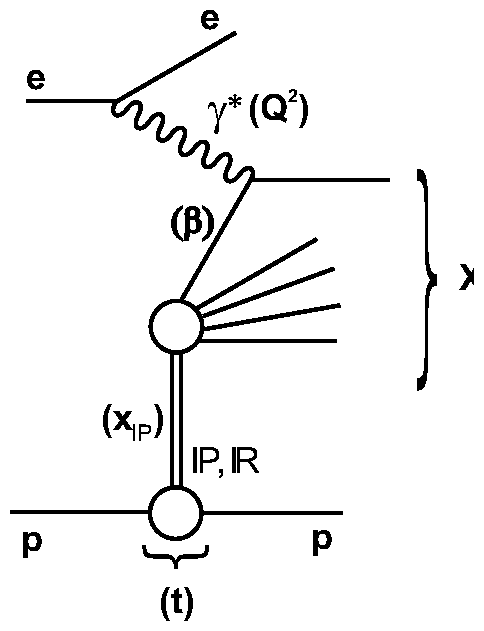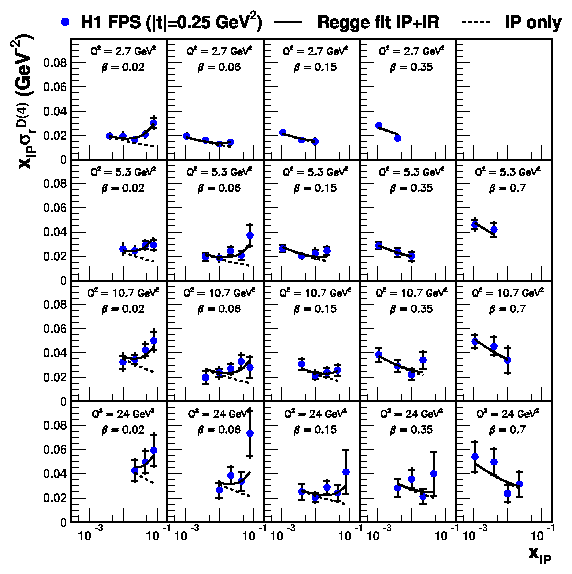# Diffractive Deep-Inelastic Scattering with a Leading Proton at HERA

The above paper presents a measurement of the diffractive deep-inelastic scattering (DIS) cross section with the H1 detector at HERA. The process ep→eXp is studied by tagging the leading final state proton. The Forward Proton Spectrometer (FPS) is used to detect and measure the four-momentum of the outgoing proton.  Forward protons scattered through small angles are deflected by the proton beam-line magnets into a system of detectors placed within the proton beam pipe inside movable stations known as Roman Pots. This detection method has the advantages that the proton unambiguously scatters elastically and that the squared four-momentum transfer at the proton vertex t can be reconstructed. However, the available statistics are limited by the FPS acceptance.The FPS data provide a means of studying inclusive diffraction as a function of all relevant kinematic variables. In addition to t and the usual DIS variables x and Q2, the measurements are made as a function of the fractional proton longitudinal momentum loss xIP and of β=x/xIP, which corresponds to the fraction of the exchanged momentum which is carried by the quark coupling to the virtual photon. The diffractive DIS data  have been interpreted in a combined framework, which applies the QCD factorization theorem to x and Q2 dependences and uses a Regge inspired approach to express the dependences on t and  xIP. The dependences on xIP and t can then be expressed in terms of an 'effective flux' of colorless exchange, whilst the β and Q2 dependences can be interpreted in terms of diffractive parton distribution functions (DPDFs), which describe the partonic structure of that exchange. Within Regge phenomenology, diffractive cross sections are described by the exchange of a leading pomeron (IP) trajectory αIP(t)=αIP(0)+α'IPt with an intercept αIP(0)>1. In order to describe the data at larger xIP, it is necessary to include a sub-leading exchange trajectory (IR) with an intercept αIP(0)~0.5.
The t dependence of diffractive cross sections are commonly parameterized with an exponential, dσ/dt~eBt. The values of B resulting from such fits are shown as a function of xIP in the figure.Atlow xIP , the data are compatible with a constant slope parameter, B ≈ 6 GeV2. In a Regge approach with a single linear exchanged pomeron trajectory the slope parameter B decreases with increasing xIP if α'IP>0 ('shrinkage' of the diffractive cone). The low xIP data thus favor a small value of α'IP≈ 0.06 GeV-2 , which is lower than α'IP≈ 0.25 obtained from soft hadronic interactions. Further analysis in which the slope B is allowed to vary with β and Q2 shows no significant dependences, confirming factorization of the t dependence in the proton vertex for the present data.

The results of the FPS method are compared in detail with the measurement of diffractive processes selected on the basis of a large rapidity gap (LRG) in the distribution of the final state hadrons. The measured LRG process is ep→eXY where Y corresponds to proton dissociation state with mass MY<1.6 GeV. The ratio of the LRG to FPS cross sections is found to be independent on variables  xIP, β and Q2 supporting factorization of the MY dependence in the proton vertex.In this paper diffractive reduced cross section σrD(4),Q2,xIP,t) is measured at |t|=0.25 GeV2.  The xIP dependence  is described using a model which is motivated by Regge phenomenology, in which a leading pomeron and a sub-leading exchange contribute. The effective pomeron intercept describing the data is αIP(0)~1.11, which is consistent within the errors with the value αIP(0)~1.08 obtained from the energy dependence of the total cross section. The data are also analyzed in terms of the diffractive reduced cross section σrD(3)  obtained by integrating σrD(4) over t. At fixed xIP, a relatively flat β dependence is observed over most of the kinematic range. The  Q2 dependence displays positive scaling violations, except at the highest  β~0.7. The β and Q2 behavior of  the reduced cross section σrD(3) is well described using DPDFs extracted from a next-to-leading QCD fit to LRG data.

In general, The FPS and LRG data exhibit a remarkable consistency with 'proton vertex' factorization, whereby the dependences on variables xIP , t and MY are completely independent of the variables β and Q2 , which describe the hard interaction with the photon.